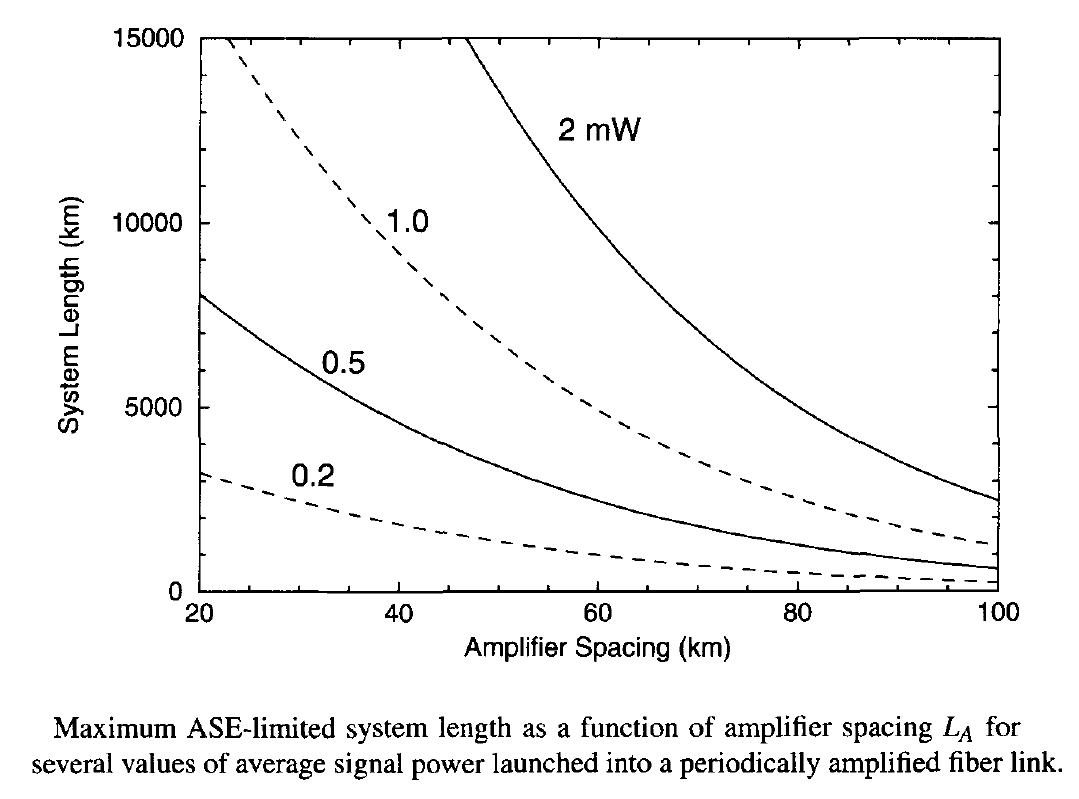# Electrical Signal-To-Noise Ratio (SNR)

Optical SNR, although useful for design purposes, is not what governs the BER at the receiver. In this tutorial, we focus on the electrical SNR of the current generated when an ASE-degraded optical signal is incident on a photodetector. For simplicity of discussion, we use the configuration shown in the figure below and assume that a single optical amplifier is used before the receiver to amplify a low-power signal before it is detected. This configuration is sometimes used to improve the receiver sensitivity through optical pre-amplification.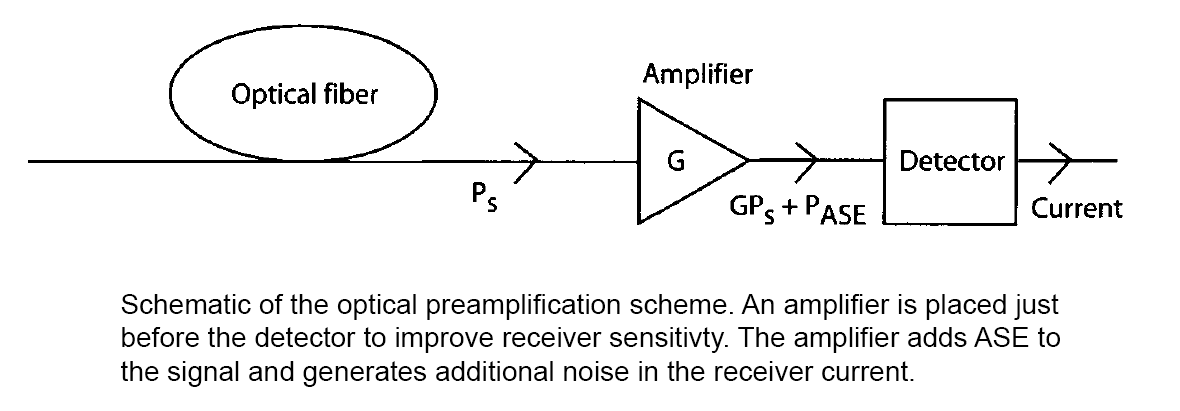#### 1. ASE-Induced Current Fluctuations

When the contribution of ASE noise to the signal field Es is included, the photocurrent generated at the receiver can be written aswhere G is the amplifier gain, is and iT are the current fluctuations induced by the shot noise and thermal noise, respectively, Ecp is the part of the ASE copolarized with the signal, and Eop is the orthogonally polarized part of the ASE. It is necessary to separate the ASE into two parts because only the copolarized part of the ASE can beat with the signal.

The ASE-induced current noise has its origin in the beating of Es with Ecp and the beating of ASE with itself. To understand this beating phenomenon more clearly, notice that the ASE occurs over a broader bandwidth than the signal bandwidth Δνs. It is useful to divide the ASE bandwidth Δνo into M bins, each of bandwidth Δνs, and write Ecp in the formwhere φm is the phase of the noise component at the frequency ωm = ωl + m(2πΔνs) and ωl is the lower boundary of the filter passband. The spectral density of ASE for a lumped amplifier is given byA form identical to that of Ecp above applies for Eop.

Usingand Ecp expression above and including all beating terms, the current I can be written in the form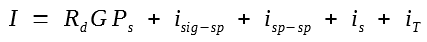where isig - sp and isp - sp represent current fluctuations resulting from signal-ASE and ASE-ASE beating, respectively, and are given by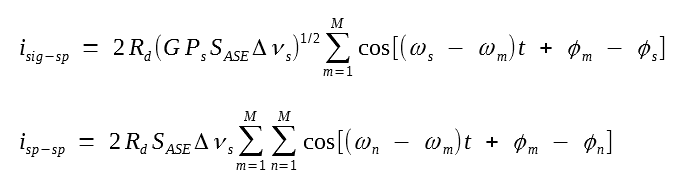since these two noise currents fluctuate rapidly with time, we need to find their averages and variances. It is easy to see that ⟨isig - sp⟩ vanishes. However, ⟨isp - sp has a finite value resulting from the terms in the double sum for which m = n. This average value is given byVariances of the two noise currents can also be calculated by squaring them and averaging over time. We write the final result directly here: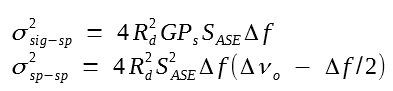where Δf is the effective noise bandwidth of the receiver. The total variance σ2 of current fluctuations can be written as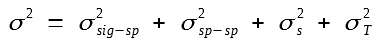where σT2 is due to thermal noise and the shot-noise variance has an additional contribution resulting from the average, that is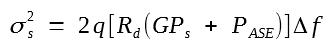where PASE = 2SASEΔνo is the total ASE power entering the receiver.

#### 2. Impact of ASE on SNR

We can now calculate the electrical SNR at the receiver. Noting that ⟨I⟩ = Rd(GPs + PASE), the SNR is given by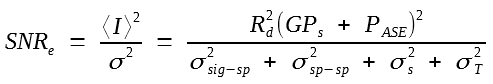The important question is whether the SNRe is improved or degraded because of signal amplification before its detection. To answer this question, we compare the above equation with the SNR realized in the absence of optical amplifier. Setting G = 1 and PASE = 0, this SNR is given by isig - sp2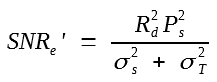Consider first the case of an ideal receiver with no thermal noise and 100% quantum efficiency so that Rd = q/hν0. In this case, SNR without an amplifier is given by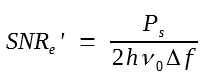When an optical amplifier is used, the current variance is dominated by σ2sig - sp. Neglecting σ2sp - sp and PASE in SNRe equation above, the SNR is found to be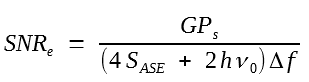Usingthe noise figure of an optical amplifier is found to be given by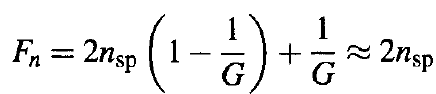In practice, thermal noise exceeds shot noise by a large amount, and it should be included before concluding that an optical amplifier always degrades SNRe. Neglecting shot noise in SNRe' expression above and retaining only the dominant term σ2sig - sp in SNRe expression, we find that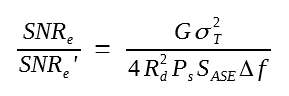As this ratio can be made quite large by lowering Ps and increasing the amplifier gain G, electrical SNR can be improved by 20 dB or more compared with its value possible without amplification. This apparent contradiction can be understood by noting that the receiver noise, dominated by σ2sig - sp, is so large in magnitude that thermal noise can be neglected in comparison to it. In other words, optical pre-amplification of the signal helps to mask the thermal noise, resulting in an improved SNR. In fact, if we retain only the dominant noise term, the electrical SNR of the amplified signal becomes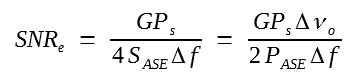This should be compared with the optical SNR of GPs/PASE. As seen in the equation above, electrical SNR is higher by a factor of Δνo/(2Δf) under identical conditions since ASE noise contributes only over the receiver bandwidth Δf that is much narrower than the filter bandwidth Δνo.

#### 3. Noise Buildup in an Amplifier Chain

As mentioned earlier, long-haul lightwave systems require cascading of multiple optical amplifiers in a periodic fashion. The buildup of ASE noise is the most critical factor for such systems for two reasons. First, in a cascaded chain of optical amplifiers, the ASE accumulates over many amplifiers and degrades the SNR as the number of amplifiers increases. Second, as the level of ASE grows, it begins to saturate optical amplifiers and reduce the gain of amplifiers located further down the fiber link. The net result is that the signal level drops while the ASE level increases. Numerical simulations show that the system is self-regulating in the sense that the total power obtained by adding the signal and ASE powers (PTOT = Ps + PASE) remains relatively constant. The figure below shows this self-regulating behavior for a cascaded chain of 100 amplifiers with 100-km spacing and 35-dB small-signal gain. The power launched by the transmitter is 1 mW. The other parameters are nsp = 1.3 and G0exp(- αLA) = 3. The signal and ASE powers become comparable after 10,000 km. Clearly, ASE-induced gain saturation should be avoided as much as possible. We assume this to be the case in the following discussion.To estimate the SNR associated with a long-haul lightwave system, we assume that all amplifiers are spaced apart by a constant distance LA, and the amplifier gain G ≡ exp(αLA) is just large enough to compensate for fiber losses in each fiber section. The total ASE power for a chain of NA amplifiers is then given by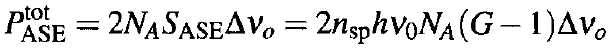and can be used to find the optical SNR using SNRo = Pin/PASE. To calculate the electrical SNR, we need to find the current variance at the receiver. If we assume that the receiver noise is dominated by the signal-ASE beat noise and include only this dominant contribution, the electrical SNR is found to be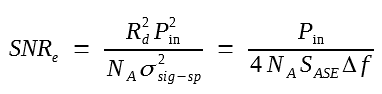This equation shows that the electrical SNR is reduced by a factor of NA simply because ASE noise is increased by that factor. However, one should not conclude from this equation that the system performance can be improved by placing fewer amplifiers along the link. As discussed before, if we reduce the number of amplifiers, each amplifier would add more noise because it has to operate with a higher gain. The results shown in the figure below also apply here because the optical and electrical SNRs are related to each other as SNRe/SNRo = Δνo/(2Δf).